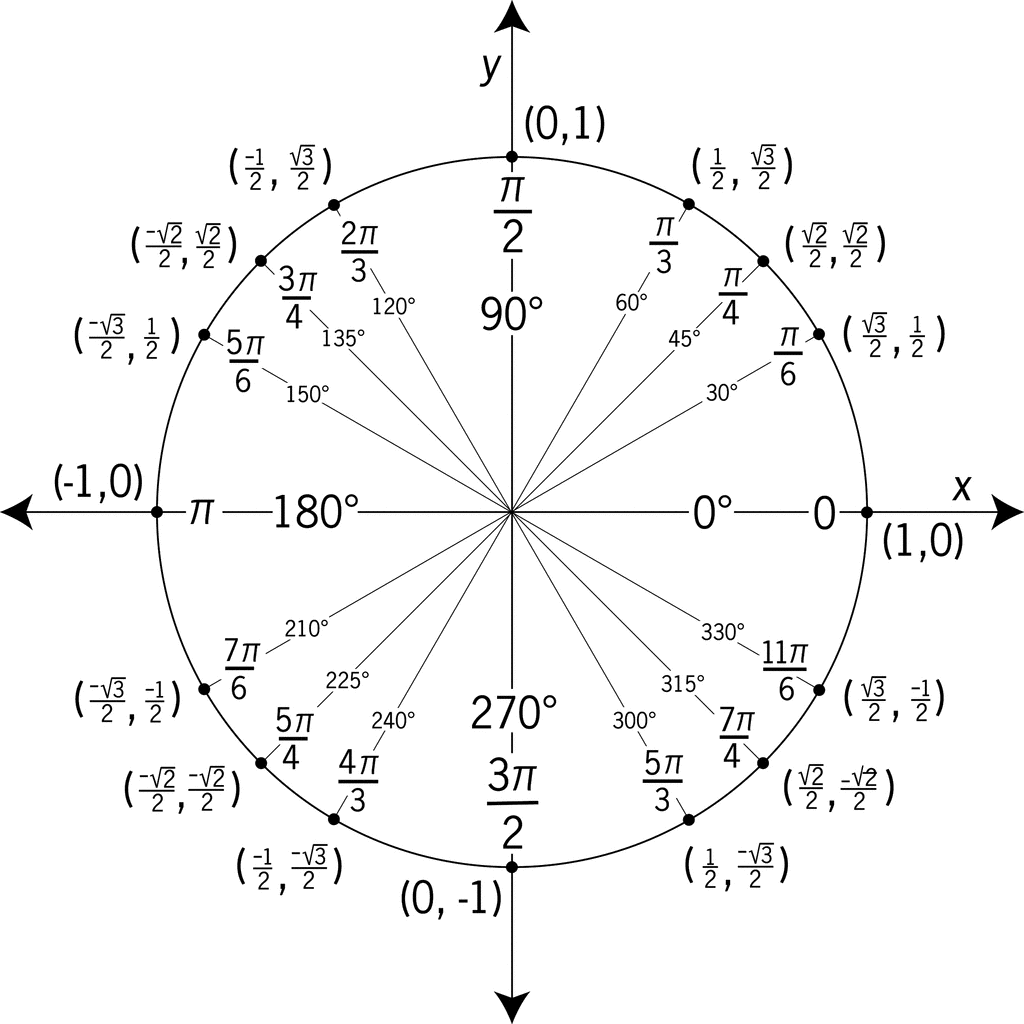# What is a Unit Circle?

A unit circle is a circle on the Cartesian Plane that has a radius of 1 unit and is centered at the origin (0, 0). The unit circle is a powerful tool that provides us with easier reference when we work with trigonometric functions and angle measurements.You can apply the Pythagorean Theorem to the unit circle. Recall, that we use the Pythagorean Theorem to find a missing side of a right triangle.

#### The Pythagorean Theorem: a2 + b2 = c2

In the image of the unit circle to the right, the triangle created is a right triangle. The side lengths of x and y are the legs where the radius (r) is the hypotenuse.

Using the Pythagorean Theorem we have:

x2 + y2 = 12 --> x2 + y2 = 1

## Trigonometric Functions and the UnitCircle

The unit circle can be used to help us generalize trigonometric functions.

If we let the x = cos θ and y = sin θ,  then P will be the terminal side of angle θ where it intersects the unit circle.

We can use this to find Sine andCosine given a point on the unit circle.

#### Unit Circle - Example Problem 1:

The terminal side of angle θ in standard position intersects the unit circle at

Find cos θ and sin θ.

#### Unit Circle - Example Problem 2:

The terminal side of angle θ in standard position intersects the unit circle at P(0,1). Find cos θ and sin θ.

Answer: P(0,1) = P(cos θ, sin θ), cos θ = 0 and sin θ = 1

The unit circle below can be used to find the exact values of cos θ and sin θ for special angles regardless of if you are working in degrees or radians. As you can see from the unit circle, the degrees range from 0o to 360o and the radians from 1 to 2π. If you just remember that P(cos θ, sin θ), then you can find a specific value.

#### Unit Circle - Example Problem 3:

Find the exact value of the expression, cos (5π/6),

On the unit circle above, locate the reference radian 5π/6 . Since P(cos θ, sin θ) and the reference point of the radian is

#### Unit Circle - Example Problem 4:

Find the exact value of the expression, sin (180o).

On the unit circle above, locate the reference degree 180o. Since P(cos θ, sin θ) and the reference point of the degree is (-1,0), sin (180o) = 0.

If you know P(cos θ, sin θ), then you can also find the exact value of the tangent function.

Recall, that tan θ = sin θ / cos θ .

#### Unit Circle - Example Problem 5:

Find the exact value of the expression, tan ( π / 4 ).